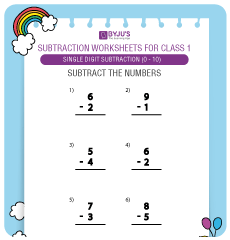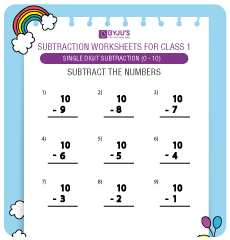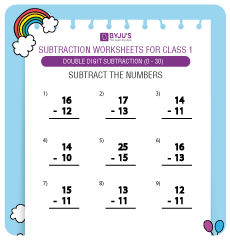# Compelling Maths Subtraction Worksheets for Grade 1 KidsHere we shall explore the Maths Subtraction Worksheets for Grade 1 kids. Practising these Subtraction activities for Grade 1 will definitely help children in understanding the arithmetic operation of Subtraction for Class 1 kids.

One of the most important operations of arithmetic is subtraction. The action of removing or minimizing numbers from a collection of numbers is referred to as Subtraction. Subtraction is considered as one of the key topics of Class 1 Maths subject.

We bring you Maths Subtraction Worksheets for Grade 1 to help your child in practising simple problems on subtraction like single-digit subtraction, missing numbers, double-digit numbers subtraction, etc.

Children are taught to learn simple subtraction sums for Class 1 like subtracting single-digit numbers and practice subtraction problems with whole numbers from 0 to 10. Subtraction is often referred to as “minus” in mathematical language.##### Single Digit Subtraction Worksheets

Solve this Maths Subtraction Worksheet for Class 1 where kids have to perform single digit subtraction i.e. subtract a single digit from another single digit.##### Single Digit Subtraction Worksheets

In this Maths Subtraction Worksheet for Class 1, kids are supposed to subtract single-digit numbers from the double-digit number “10”.##### Double Digit Subtraction worksheet

In this simple Subtraction Worksheet for Class 1, students have to perform double-digit subtraction, i.e. subtract a double-digit from another double digit number.

In Class 1 Maths, children learn to solve subtraction mental problems like subtracting double-digit numbers or single-digit numbers, missing numbers, subtracting in columns, etc. As your child begins learning Maths, this is the beginner stage of learning Subtraction sums for Class 1 since this prepares your child for higher grades.

Solving the above Subtraction Worksheets for 1st Grade opens up the kids’ minds and boosts their confidence in the subject. You may want to explore various other Maths Worksheets for Class 1 here

Our simple Subtraction Worksheets for Grade 1 are designed in a simple manner that will help your child to understand the Subtraction for Class 1 and be ready to solve any single-digit or double-digit subtraction problems easily. Subtraction sums for Class 1 provided here are designed in a manner that entices kids and engages them in solving these sums. Do you wish to explore more such interesting kids education resources for your little ones? Check out BYJU’S Kids Learning section and get access to the most interesting resources for your children.